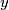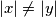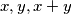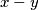### IMO Shortlist 1999 problem C6

Kvaliteta:
Avg: 0,0
Težina:
Avg: 8,0
Suppose that every integer has been given one of the colours red, blue, green or yellow. Let$x$ and$y$ be odd integers so that$|x| \neq |y|$. Show that there are two integers of the same colour whose difference has one of the following values:$x,y,x+y$ or$x-y$.
Izvor: Međunarodna matematička olimpijada, shortlist 1999